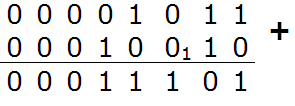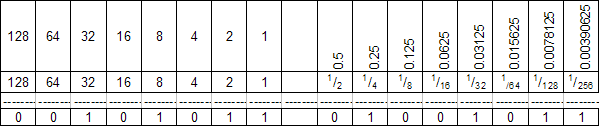# Computer ScienceBinary Numbers

## Denary Numbers

Our number system is called the denary or base 10 system. 10 digits (0 - 9) are used for counting.

You should remember the place value table from your Maths lessons in Year 7.

Eg 47395

10000000100000010000010000100010010Units
00047395

As each place value moves one to the left, the power of ten is increased by 1. The units column is 100, the tens, 101, the hundreds, 102 and so on.

## Binary Numbers

The place value columns in the binary table below are powers of 2. The only valid digits to use are 1 and 0. We call these bits. Just like in the denary table, we add up the results of multiplying each number in the table by its place value. Click on the buttons below the table to see how the numbers from 0 to 255 can be represented in pure binary. The denary value is shown below the table.

128643216842Units
00000000
0

Addition using binary numbers is relatively straightforward. The following rules need to be observed,

• 0 + 0 = 0
• 0 + 1 = 1 + 0 = 1
• 1 + 1 = 10 (0, carry 1)
• 1 + 1 + 1 = 11 (1, carry 1)

In the following example, the binary numbers, 1011 (11 in denary) and 10010 (18 in denary) are added together.When performing binary addition, you must lay your sums out this way. Do not work things out in your head or use any mental methods that you learned in little school. The potential for error is too great not to take care with something that is quite basic.

## Representing Negative Numbers

The number shown in the interactive binary place value table is an unsigned binary integer. That means that it is a positive whole number as far as we are concerned.

In denary, when we want to represent a negative number, we simply place a minus sign before it. Binary doesn't work that way.

The Two's Complement system is used to represent negative numbers in binary. The system works a bit like a milometer. If the milometer is set at 00000 and is turned back one mile, it would read 99999. A negative binary number always has a 1 as the first bit. This is often referred to as the sign bit

## Convert From Denary To Two's Complement

To convert a negative denary number to binary, first find the binary equivalent of the positive integer.

Eg -27, 27 = 00011011

Change all of the 0s to 1s and all the 1s to 0s. (Flip the bits).

Eg 11100100

Eg 11100101

The following place value table works like the previous one. This one allows you to show negative numbers. Pick a random negative number. Follow the steps described above for converting to two's complement. You should end up with the positive number.

-128643216842Units
00000000
0

## Convert From Two's Complement To Denary

The Two's Complement method of representing negative numbers makes sense if you think of the sign bit as representing a negative number. In the case of the binary counter above, the first column represents -128. Convert to denary as normal, adding the column heading whenever there is a 1 below it.

## Binary Subtraction

To perform subtraction in binary, simply convert the number that you are subtracting into Two's Complement form and add it to the other number. You do not carry the one on the leftmost digit.

## Key Points To Remember

• A positive number always has 0 as the Most Significant Bit.
• A negative number always has 1 as the Most Significant Bit.
• An even number always has 0 as the Least Significant Bit.
• An odd number always has 1 as the Least Significant Bit.
• The number of digits used to represent a number is called the word length.
• -1 is always represented with a 1 in every bit.

## Fixed Point Binary Numbers

So far, you have only looked at ways of representing binary integers. To understand how binary fractions work, first consider how decimal fractions work.

100010010Units 1/101/1001/100
0549.367

In binary, the column headings are powers of 2 rather than 10.

842Units 1/21/41/81/16
1011.1011

This would store the number 8 + 2 + 1 + 0.5 +0.125 + 0.0625 = 11.6875

When storing a fraction in binary digits the binary point is not stored. We need to assume a certain number of bits before the binary point and a number after the binary point. This representation of binary numbers is called fixed point binary.

Assuming 8 bits either side of the binary point and we get the following headings,In the above example the number stored would be :

32 + 8 + 2 + 1 + 0.25 + 0.03125 + 0.0078125 + 0.00390625 = 43.2869375

Unless a number is an exact power of 2 it is impossible to store it exactly using this method. Errors in conversion of decimal numbers arise unless a large number of bits are allocated to store them.

You can convert any decimal fraction to a binary fraction. Multiply the fractional part of the number by 2. Take the integer part of the result (1 or 0) as the first bit. Repeat this process with the result until you run out of patience. For example, to convert 0.3568 into fixed point binary with 8 bits to the right of the binary point,

0.3568 x 2 = 0.7136 :0
0.7136 x 2 = 1.4272 :1
0.4272 x 2 = 0.8544 :0
0.8544 x 2 = 1.7088 :1
0.7088 x 2 = 1.4176 :1
0.4176 x 2 = 0.8352 :0
0.8352 x 2 = 1.6704 :1
0.6704 x 2 = 1.3408 :1

0.3568 is .01011011

When we convert the binary result back to denary, we get 0.35546875. This isn't too far away - with 16 bits we could get, 0.356796264648437. The precision increases the more bits we use.